Indirect questions - finding remaining observations

Chapter 13 Class 11 Statistics
Concept wise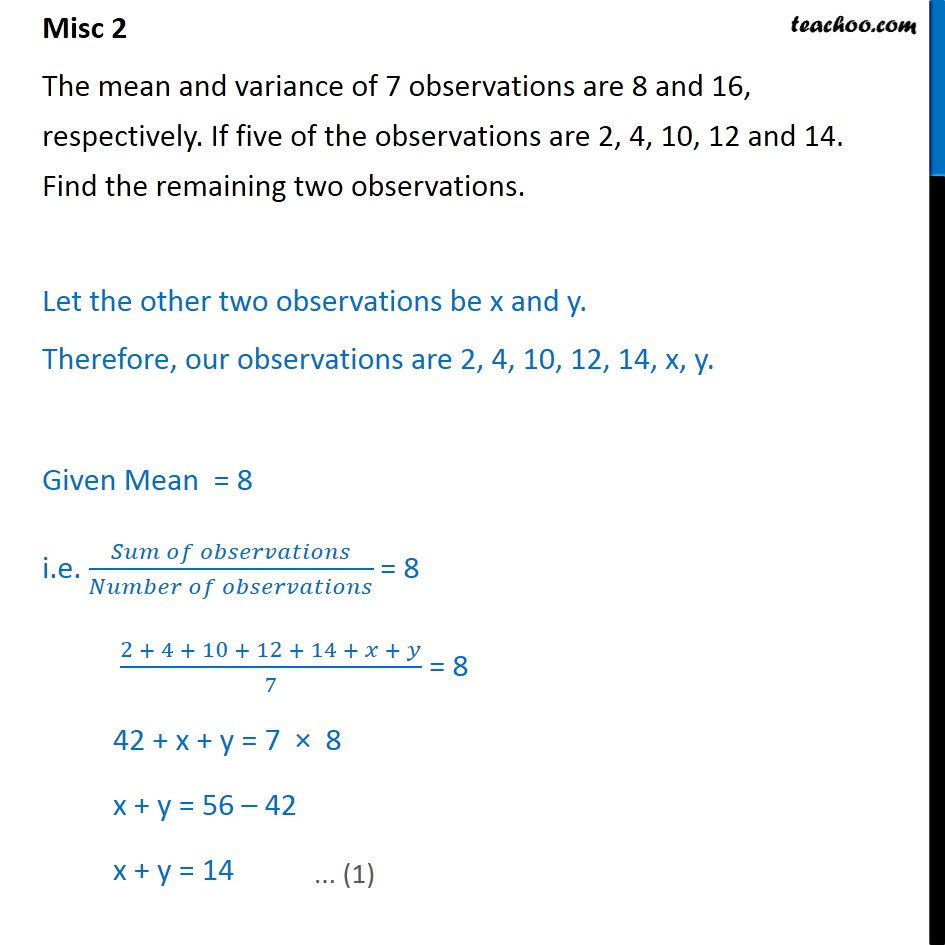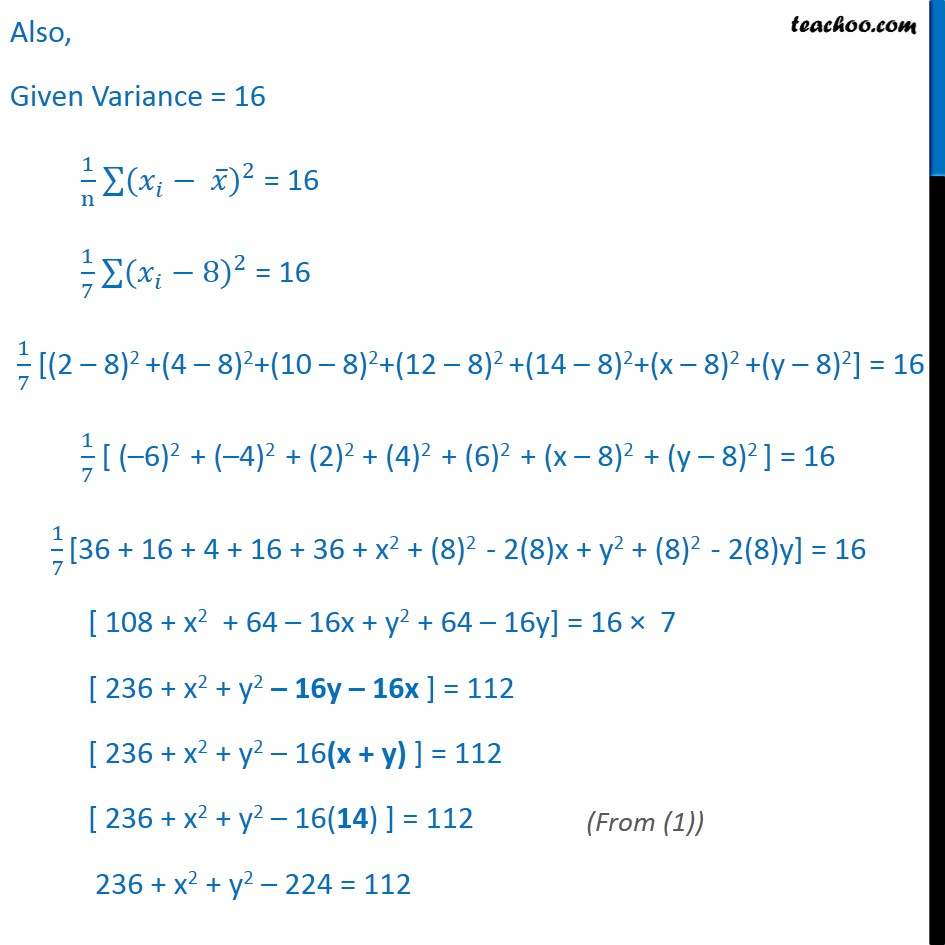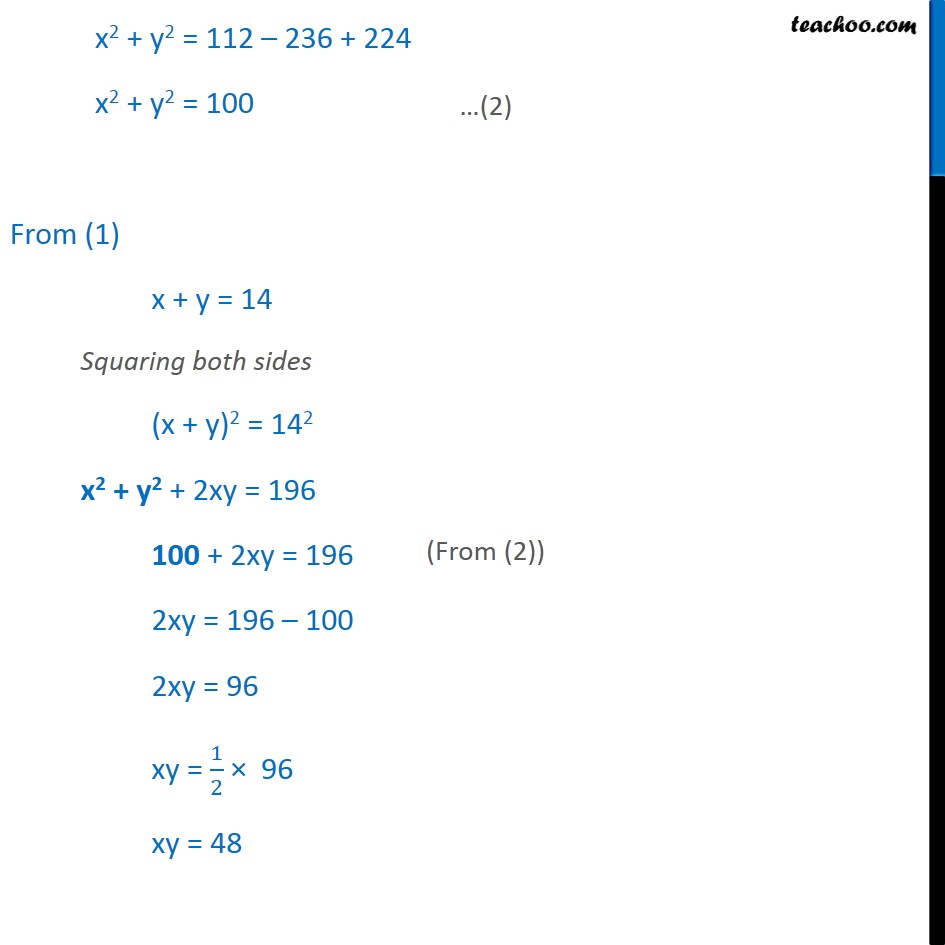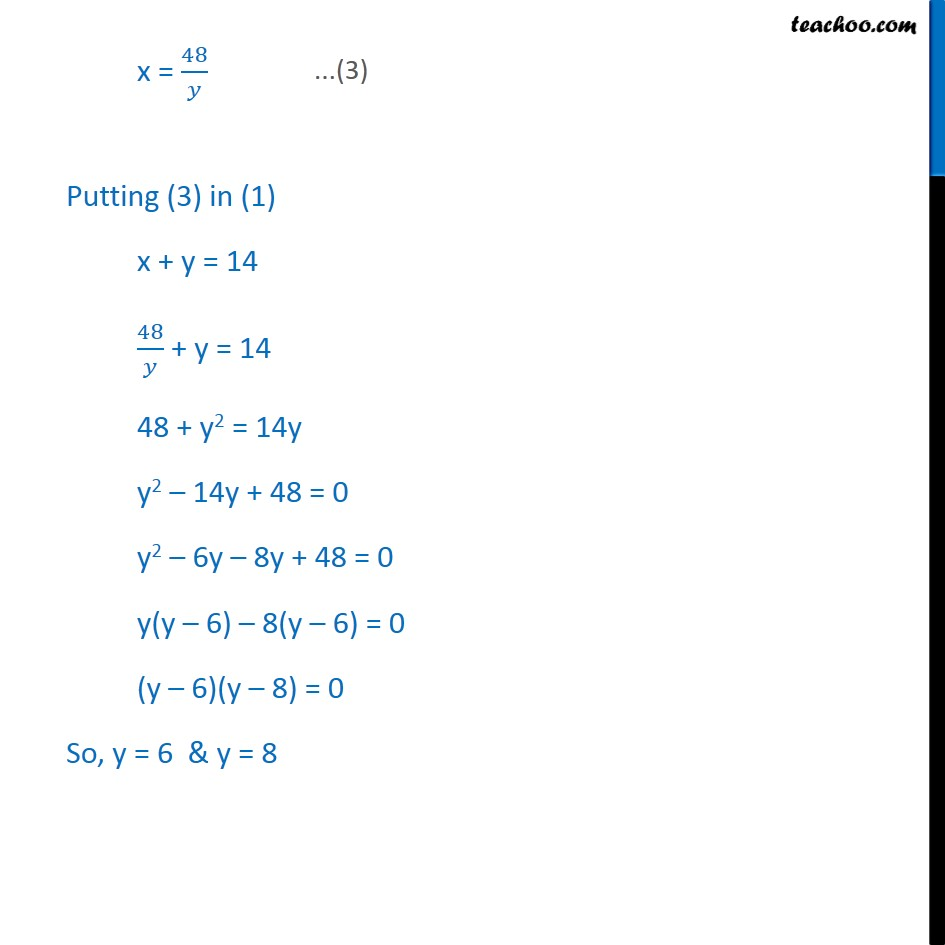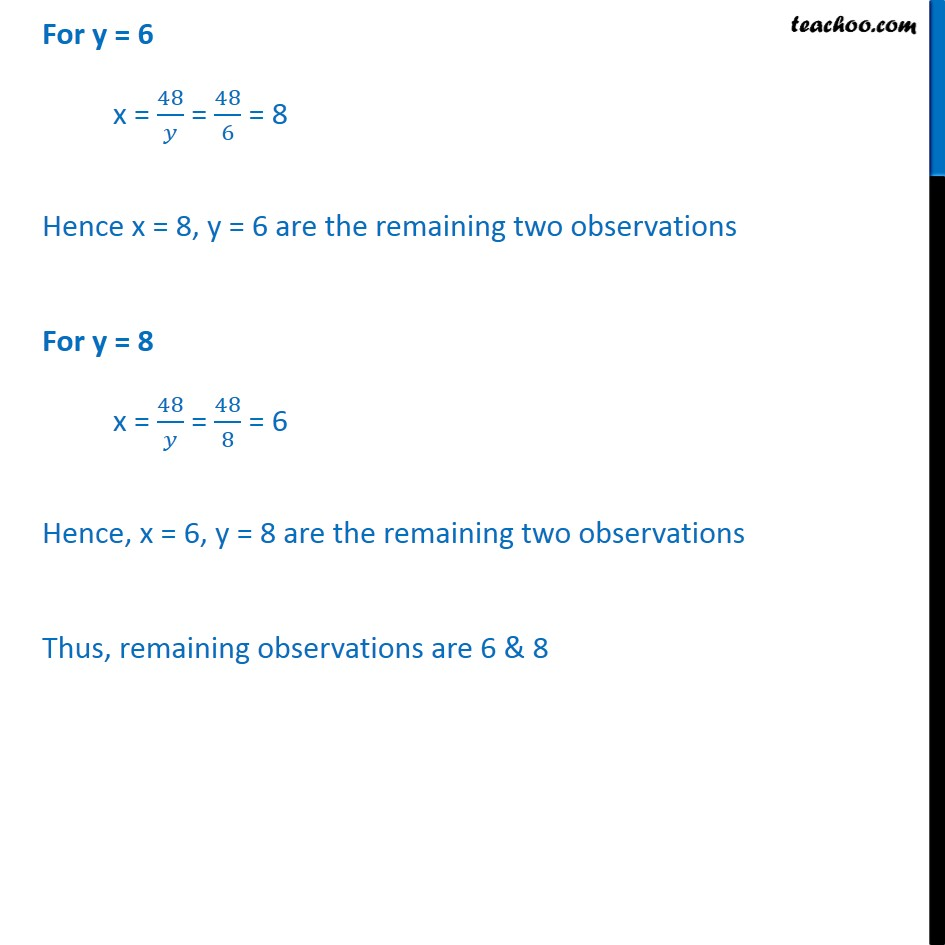Learn in your speed, with individual attention - Teachoo Maths 1-on-1 Class

### Transcript

Misc 2 The mean and variance of 7 observations are 8 and 16, respectively. If five of the observations are 2, 4, 10, 12 and 14. Find the remaining two observations. Let the other two observations be x and y. Therefore, our observations are 2, 4, 10, 12, 14, x, y. Given Mean = 8 i.e. 𝑆𝑢𝑚 𝑜𝑓 𝑜𝑏𝑠𝑒𝑟𝑣𝑎𝑡𝑖𝑜𝑛𝑠﷮𝑁𝑢𝑚𝑏𝑒𝑟 𝑜𝑓 𝑜𝑏𝑠𝑒𝑟𝑣𝑎𝑡𝑖𝑜𝑛𝑠﷯ = 8 2 + 4 + 10 + 12 + 14 + 𝑥 + 𝑦﷮7﷯ = 8 42 + x + y = 7 × 8 x + y = 56 – 42 x + y = 14 Also, Given Variance = 16 1﷮n﷯ ﷮﷮( 𝑥﷮𝑖﷯﷯− 𝑥﷯)﷮2﷯ = 16 1﷮7﷯ ﷮﷮( 𝑥﷮𝑖﷯﷯−8)﷮2﷯ = 16 1﷮7﷯ [(2 – 8)2 +(4 – 8)2+(10 – 8)2+(12 – 8)2 +(14 – 8)2+(x – 8)2 +(y – 8)2] = 16 1﷮7﷯ [ (–6)2 + (–4)2 + (2)2 + (4)2 + (6)2 + (x – 8)2 + (y – 8)2 ] = 16 1﷮7﷯ [36 + 16 + 4 + 16 + 36 + x2 + (8)2 - 2(8)x + y2 + (8)2 - 2(8)y] = 16 [ 108 + x2 + 64 – 16x + y2 + 64 – 16y] = 16 × 7 [ 236 + x2 + y2 – 16y – 16x ] = 112 [ 236 + x2 + y2 – 16(x + y) ] = 112 [ 236 + x2 + y2 – 16(14) ] = 112 236 + x2 + y2 – 224 = 112 x2 + y2 = 112 – 236 + 224 x2 + y2 = 100 From (1) x + y = 14 Squaring both sides (x + y)2 = 142 x2 + y2 + 2xy = 196 100 + 2xy = 196 2xy = 196 – 100 2xy = 96 xy = 1﷮2﷯ × 96 xy = 48 x = 48﷮𝑦﷯ Putting (3) in (1) x + y = 14 48﷮𝑦﷯ + y = 14 48 + y2 = 14y y2 – 14y + 48 = 0 y2 – 6y – 8y + 48 = 0 y(y – 6) – 8(y – 6) = 0 (y – 6)(y – 8) = 0 So, y = 6 & y = 8 For y = 6 x = 48﷮𝑦﷯ = 48﷮6﷯ = 8 Hence x = 8, y = 6 are the remaining two observations For y = 8 x = 48﷮𝑦﷯ = 48﷮8﷯ = 6 Hence, x = 6, y = 8 are the remaining two observations Thus, remaining observations are 6 & 8